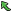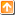## 4102: Identity MatrixTime Limit(Common/Java):1000MS/3000MS     Memory Limit:65536KByte
Total Submit: 318            Accepted:101

Description

In linear algebra, the identity matrix or unit matrix of size n is the n×n square matrix with ones on the main diagonal and zeros elsewhere.
Identity Matrix is denoted with the letter “In×n”, where n×n represents the order of the matrix. One of the important properties of identity matrix is: A×In×n = A, where A is any square matrix of order n×n.

Given an n×m matrix, if it is an identity matrix, please output "Yes", or else output "No".

Input

There are multiple cases. In each case, the first line has two integers n and m (1<=n, m<=10), then follows n lines, each line has m integers.

The input is ended with n=0 and m=0.

Output

Each case outputs "Yes" or "No" in one line where the quotation marks are excluded.

Sample Input

Sample Output

Source

Ě¨ÖÝŃ§ÔşµÚËÄ˝ěĐÂÉúłĚĐňÉčĽĆľşČü

|Back |   |Top|